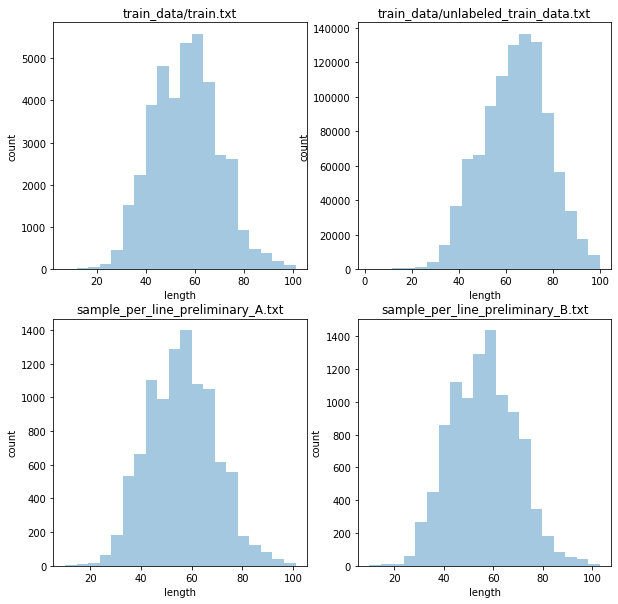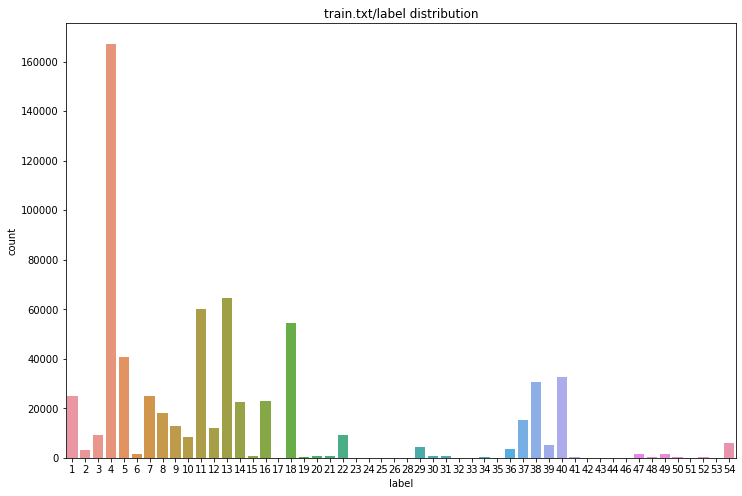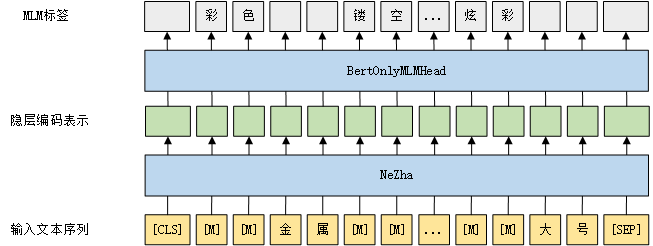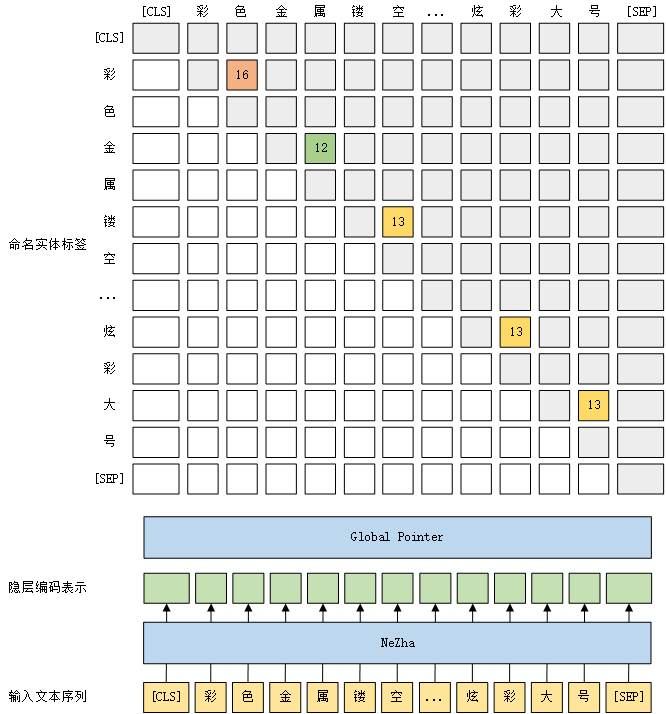## 赛题介绍

• 训练数据包含4W条有标注样本和100W条无标注样本，选手可自行设计合理的方案使用；
• 初赛A榜、B榜分别公开1W条测试集样本，可下载到本地用于模型训练（如，作为预训练语料、用作伪标签数据）；
• 复赛阶段测试集同样也是1W条，但只能在线上推理时根据路径读取，无法下载到本地。

\begin{aligned} P &= \frac{|S \bigcap G|}{|S|} \\ R &= \frac{|S \bigcap G|}{|G|} \\ F_1 &= \frac{2 P R}{P + R} \\ \end{aligned}

• 模型在单卡（NVIDIA T4，或者同等算力的 GPU 卡）上单条数据的推理时间要小于360ms，如果超过360ms，会根据推理耗时进行惩罚：

• 如果模型在单卡上单条数据的平均推理时间小于360ms，不做惩罚；
• 反之，如果大于360ms，需要乘以一定的惩罚系数

具体如下：

$F_1 = \begin{cases} F_1 & \text{if} & t_{\text{inference}} \leq 360 \\ F_1 \left( 1 - \frac{t_{\text{inference}} - 360}{2000} \right) & \text{if} & t_{\text{inference}} > 360 \\ \end{cases}$

• 若超过1.5小时，线上将自动停止评审，并反馈“超过最大运行时间”。

## 数据分析• 文本以短句为主，最大长度不超过128，各数据集文本长度分布大致一致，长度主要集中在60左右；
• 除少部分实体长度过长外（217个实体长度超过20，约占总体0.03%），其余实体长度主要集中在10以内；
• 总计包含662,478个实体，存在明显的类别不均衡问题，最多的实体类别是4，占全部实体的25.25%，而24263553等类型实体数量均少于10；
• 商品标题一般由大量关键字组合而成，因此句中实体分布稠密，而且实体间没有重叠关系。

## 总体方案• 微调阶段：在预训练阶段（二）训练得到的模型基础上，用下游命名实体识别任务的标注数据微调。命名实体模型采用GlobalPointer，这是一种将文本片段头尾视作整体进行判别的命名实体识别方法，详情可参考GlobalPointer：用统一的方式处理嵌套和非嵌套NER - 科学空间。不同的是，我们采用多分类方式建模而不是多标签方式。

## 数据处理

BERT分词器和改进后的分词器对比效果如下，我们用[unused1]来代表文中的空白符：

## 模型构建

（1）预训练阶段（2）微调阶段：## 方案优化

### 数据增强

1. 随机选择token并用[MASK]替换：目的是加强模型的上下文建模能力，提高模型的泛化性；
2. 随机选择实体并用[MASK]替换：方案1的改进版，不再随机选择token，而是选择完整的实体掩盖；
3. 随机选择实体并用同义词替换：方案2的改进版，不再用[MASK]而是用实体的同义词，同义词由Word2Vec词向量确定；
4. 随机丢弃文本中的实体：随机选择完整的实体删除，由于降低了实体出现频率，过多丢弃实体可能导致模型欠拟合。

### 损失函数

$L_{\text{CE}} = - \log(P_t) = \sum_{j=1}^{\infin} \frac{1}{j} (1 - P_t)^j$

$L_{\text{Ploy-1}} = (1 + \epsilon_1)(1 - P_t) + \frac{1}{2} (1 - P_t)^2 + \cdots = L_{\text{CE}} + \epsilon_1 (1 - P_t)$

### 对抗训练

$\theta = \arg \min_{\theta} E_{(x, y) \sim \mathcal{D}} \left[ \max_{r_{adv} \in S} L (\theta, x + r_{adv}, y) \right]$

(1) FGM

$r_{adv} = \arg \max_{||r||_2 \leq \epsilon} p(y | x + r, \theta) = \epsilon \cdot \frac{g}{||g||_2}$

(2) AWP

$\min_w \max_{v \in V} \rho(w+v) \to \min_w \max_{v \in V} \frac{1}{n}\sum_{i=1}^n \max_{\parallel x^{‘}_i -x_i \parallel_p \leqslant \epsilon } \ell(f_{w+v}(x^{'}_i,y_i))$

### R-Drop1. 同一样本两次先后输入模型，由于Dropout的随机性，两次前向运算结果可以视作两个不同模型的输出，即输出分布$p_1 (y|x)$$p_2 (y|x)$
2. 用对称形式的KL散度（Symmetric Kullback-Leibler Divergence）评估两个分布的相似性：

$L^{SKL}_i = \frac{1}{2} \left[ \text{KL}( p_1(y_i | x_i) || p_2(y_i | x_i) ) + \text{KL}( p_2(y_i | x_i) || p_1(y_i | x_i) ) \right]$

1. 最终优化目标如下，$\lambda$为损失权重

$L_i = L^{CE}_i + \lambda L^{SKL}_i$

### 后处理

• 规则：通过对比验证集标签和模型输出，我们设计了以下后处理规则:
• 若两个实体发生重叠，且实体类型相同，则从中保留一个较长或较短实体，这根据实体类型决定，如类型4需要保留短实体，38则保留长实体；
• 若三个实体发生重叠，且实体类型相同，则从中保留最长的实体；
• 若三个实体发生重叠，且实体类型不同，则从中保留最短的实体；
• ……
• NMS：上述设计的规则难免产生遗漏，因此最后会用NMS算法再处理一遍，确保结果中没有实体重叠。熟悉视觉任务的同学应该对NMS不陌生，这是一种基于贪婪的算法，作用是去除冗余的目标框。在本方案中用于去除实体嵌套时，将模型输出的类别概率作为实体片段评分，依次从剩余实体中选择评分最高的实体保留，如果当前选中实体与已保留实体重叠，那么舍弃该实体。

## 后续提升方向

1. 从周星分享内容来看，伪标签有一定的提升效果，可以从伪标签方向进行提升。
2. 本赛题官方规定只能产出一个模型，那么一定程度上可以采用知识蒸馏技术将多个模型蒸馏到单个模型。
3. 简单的EDA方案可能破坏了数据的分布，可尝试其余数据增强方法，如AEDA等。